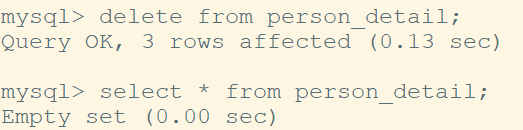# MySQL Examples

MySQL is a relational database. SQL stands for Structured query language. SQL is used to extract and write data in to MySQL. In this post, I will cover the most used commands in MySQL. Once you know these commands, there is no need to remember them. Checkout mysql autocomplete if you want to enable mysql autosuggest on mysql console.

## MySQL Create database example

Let us create a database name "person"

```CREATE DATABASE person;
```

Let us now switch to database "person"

```USE person;
```

## MySQL Drop database example

Below command will drop the database person.

```DROP DATABASE person;
```

For the rest of this post, let us re-create our database again.

```CREATE DATABASE person;
```

Let us the see databases we have now using 'show databases' command.

```show databases;
```

Ok, now we have our database created. Let us create the table using command 'create table'

## MySQL CREATE TABLE example

Following command will create table 'person_detail' with two columns with names 'name' and 'age'. Note below command will create column 'name' of type char where total length of characters could be 20 and 'age' of type INT where total integer could be upto 8 bit number.

```CREATE TABLE person_detail(name VARCHAR(20), age INT(8));
```

Let us check the data types using command 'Describe table_name'

```DESCRIBE person_detail;
```

## MySQL ALTER TABLE example

Following 'alter table' command will add column job to our table 'person_detail'

```ALTER TABLE person_detail  ADD  job varchar(20);
```

## MySQL Insert data in to table example

Following command will row of data with values 'John', 20 and 'Engineer' for the fields 'name', 'age' and 'job' respectively.

```INSERT INTO person_detail (name,age,job) VALUES('John',20,'Engineer');
```

Let us check our recently added data using select * from person_detail table;

```SELECT * FROM person_detail;
```

## MySQL CREATE INDEX example

Indexes are very important. It makes data access very fast. Indexes are really important when you have large number of rows and your table is very big.

Let us create an index on column 'name'.

```CREATE INDEX name ON person_detail (name);
```

To display indexes execute command SHOW INDEXES FROM table_name;

```SHOW INDEXES FROM person_detail;
```

## MySQL Drop INDEX example

To delete index, run command 'DROP INDEX index_name ON table_name' as shown below.

```DROP INDEX name ON person_detail;
```

## MySQL Distinct example to find unique values

Let us add following two rows.

```INSERT INTO person_detail (name,age,job) VALUES('Joe',22,'Engineer');
INSERT INTO person_detail (name,age,job) VALUES('James',42,'Doctor');
```Let us say, now we want to find all the unique values of column job. We can run distinct(column) that is distinct(job).

```SELECT DISTINCT(job) FROM person_detail;
```

## MySQL Delete rows or data example

We can delete all the data using command DELETE * FROM table_name. For example to delete the data in our table person_detail, run following command.

```DELETE * FROM person_detail;
```